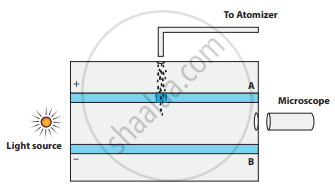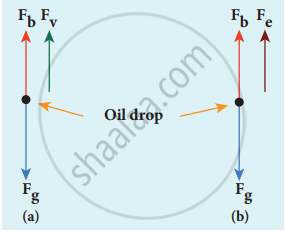Tamil Nadu Board of Secondary EducationHSC Science Class 12th

# Discuss the Millikan’s oil drop experiment to determine the charge of an electron. - Physics

Discuss the Millikan’s oil drop experiment to determine the charge of an electron.

#### Solution

Millikan’s experiment is used to determine one of the fundamental constants of nature known as a charge of an electron.

• Principle:

1. This method is based on the study of the motion of uncharged oil drop under free fall due to gravity and charged oil drop in a uniform electric field.
2. By adjusting the electric field suitably, the motion of oil drop inside the chamber can be controlled – that is, it can be made to move up or down or even kept balanced in the field of view for a sufficiently long time.
• Experimental arrangement:

1. The apparatus consists of two horizontal circular metal plates A and B each with a diameter of around 20 cm and are separated by a small distance 1.5 cm.Millikan’s experiment
2. These two parallel plates are enclosed in a chamber with glass walls. Further, plates A and B are given a high potential difference around 10 kV such that the electric field acts vertically downward.
3. A small hole is made at the centre of the upper plate A and atomizer is kept exactly above the hole to spray the liquid.
4. When a fine droplet of highly viscous liquid (like glycerine) is sprayed using an atomizer, it falls freely downward through the hole of the top plate only under the influence of gravity.
5. Few oil drops in the chamber can acquire electric charge (negative charge) because of friction with air or passage of x – rays in between the parallel plates.
6. Further the chamber is illuminated by light which is passed horizontally and oil drops can be seen clearly using a microscope placed perpendicular to the light beam. These drops can move either upwards or downward.
(i) Determination of radius of the droplet:

Fg = gravitational force
Fb = buoyant force
Fv = viscous force
viscous force and buoyant force balance the gravitational force.

7. When the electric field is switched off, the oil drop accelerates downwards. Due to the presence of air drag forces, the oil drops easily attain its terminal velocity and moves with constant velocity.Free body diagram of the oil drop – (a) without electric field (b) with electric field

8. Let the gravitational force acting on the oil drop (downward) be Fb = mg, m = mass of the oil drop g = Acceleration due to gravity.
9. Let ρ be the density of the oil drop, and r be the radius of the oil drop, then the mass of the oil drop can be expressed in terms of its density as
rho = "m"/"V"
m = rho(4/3 pi"r"^3)(volume of the sphere, V = 4/3 pi"r"^3)
10. The gravitational force can be written in terms of density as
Fg = mg
=>Fg = ρ(4/3) πr3

11. Let σ be the density of the air, the upthrust force experienced by the oil drop due to displaced air is
Fb = σ(4/3 πr3)g

12. From Stokes law, the viscous force on the oil drop is.
Fv = 6πrυη
The force balancing equation is
Fg = Fb + Fv
ρ(4/3 π"r"^3)"g" = σ(4/3 π"r"^3)"g"+ 6πrυη
4/3 πr3(ρ – σ) = 6πrυη

2/3 πr3(ρ – σ) = 3πrυη
r = [(9η"v")/(2(rho - sigma)"g")]^(1/2)   ....(1)

Thus, equation (1) gives the radius of the oil drop.

(ii) Determination of electric charge:
(a) Fe = qE
(b) buoyant force Fb

13. When the electric field is switched on, charged oil drops experience an upward electric force (qE).

14. Among many drops, one particulars drop can be chosen in the field of view of the microscope and the strength of the electric field is adjusted to make that particular drop to be stationary.

15. Under these circumstances, there will be no viscous force acting on the oil drop. Force acting on the oil droplet is
Fe + Fb = Fg
⇒ qE + 4/3 πr3 σg = 4/3 πr3 ρg
⇒ qE = 4/3 πr3 (ρ – σ)g
⇒ q = 4/(3"E") πr3 (ρ – σ)g …………..(2)

Substituting equation (1) in equation (2), we get
q = (18 pi)/"E" ((eta^3 "v"^3)/(2 (rho - sigma)"g"))^(1/2)

16. Millikan repeated this experiment several times and computed the charges on oil drops. He found that the charge of any oil drop can be written as integral multiple of a basic value, (e = 1.6 × 10-19 C), which is nothing but the charge of an electron.

Concept: Electric Discharge Through Gases
Is there an error in this question or solution?

#### APPEARS IN

Tamil Nadu Board Samacheer Kalvi Class 12th Physics Volume 1 and 2 Answers Guide
Chapter 9 Atomic and Nuclear physics
Evaluation | Q III. 2. | Page 191
Share